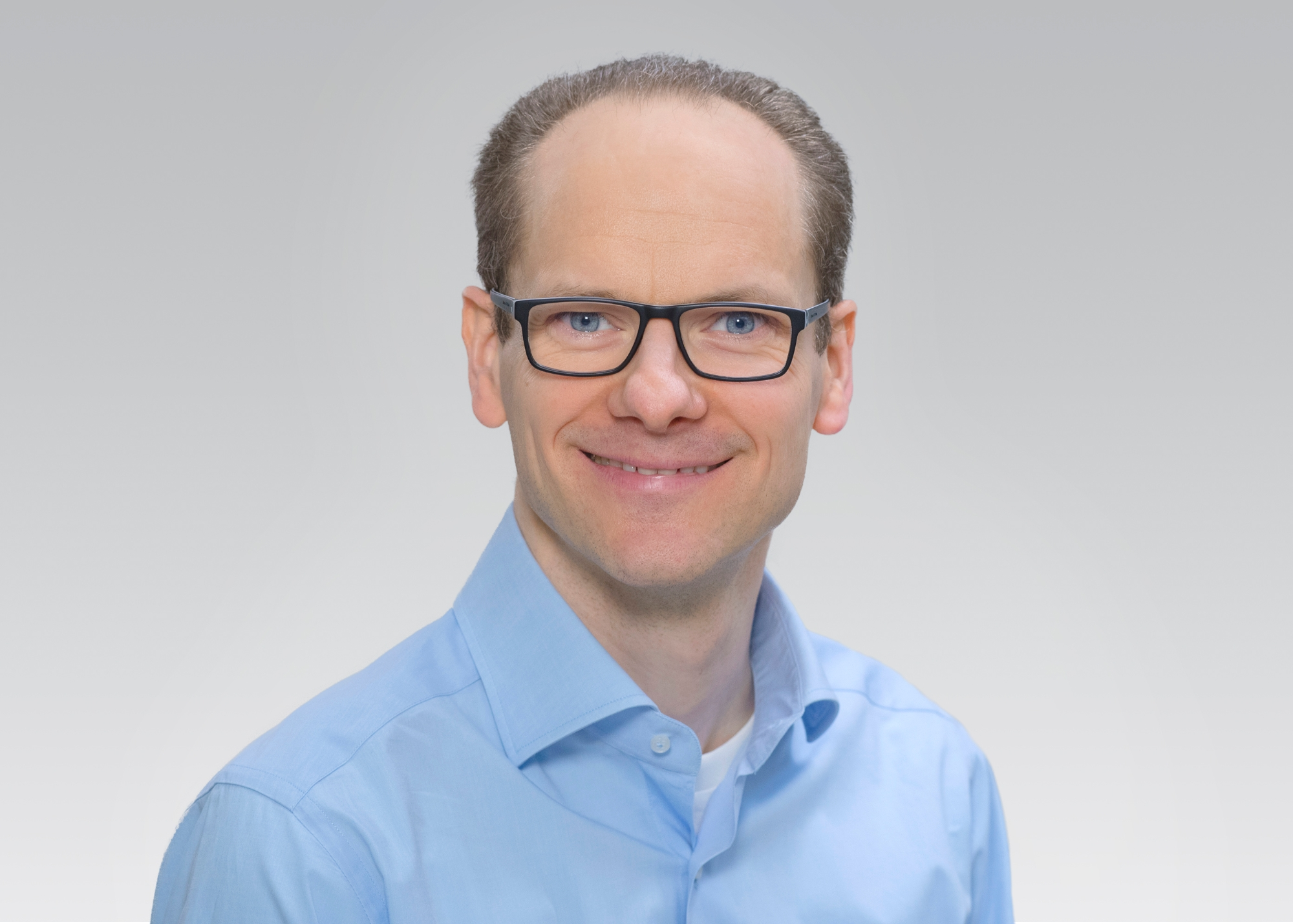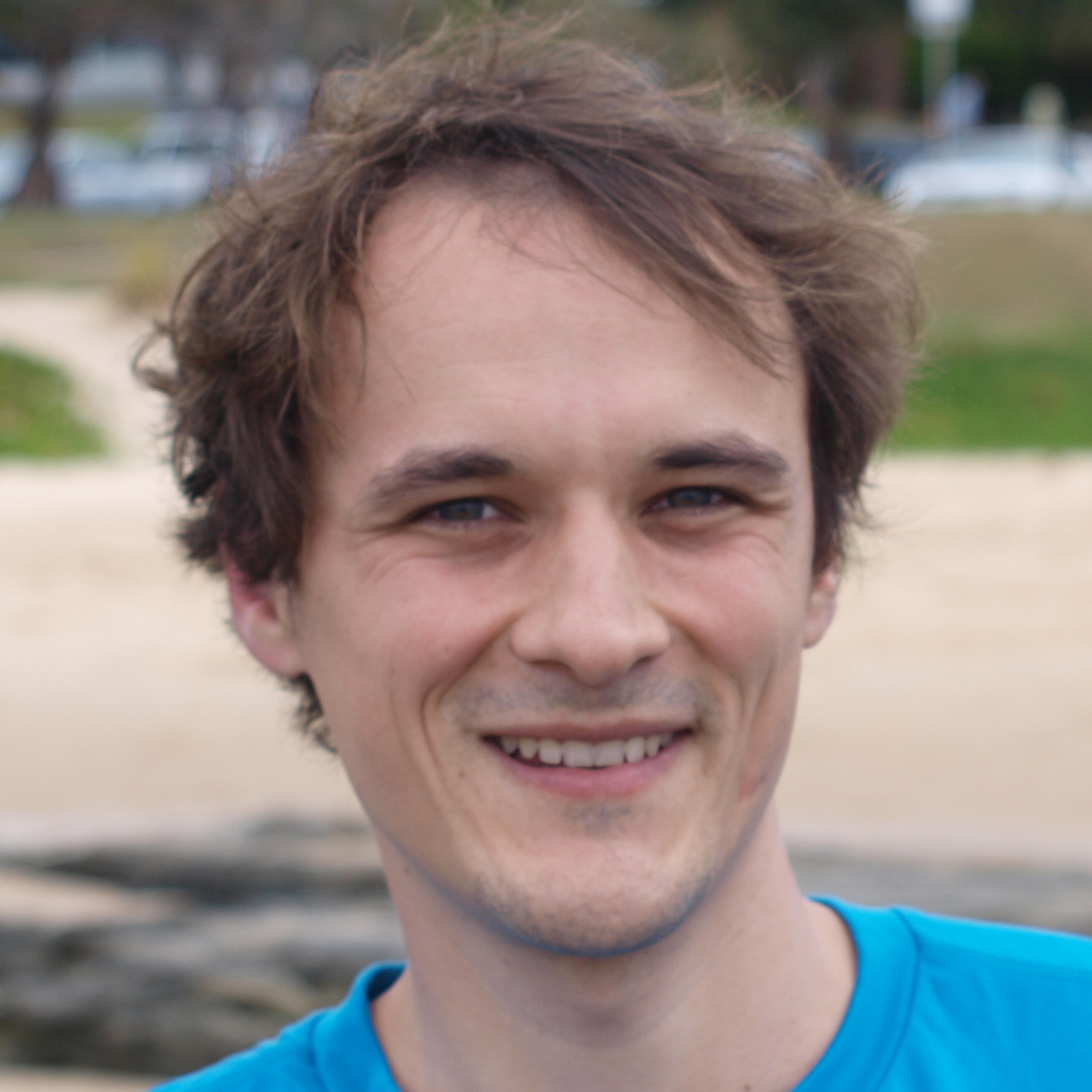# Mathematical Foundations of (Post-Quantum) Cryptography

Lecture and Exercise (Master). SWS 4 (3+1)

This lecture will introduce the mathematical foundations underlying both classical (i.e., non-post-quantum) cryptosystems as well as post-quantum secure cryptosystems. Mathematical theories used in (post-quantum) cryptography include:

• Algebra (foundations of group theory, ring theory and field/Galois theory, tensor products)
• Algebraic Number Theory (Euler’s Criterion, Fermat’s Theorem, Legendre and Jacobi symbols, number fields, algebraic lattices, discrete and fast Fourier transform)
• Algebraic Geometry (elliptic curves, Jacobi and Poincaré varieties, Weil and Tate pairing, hyperelliptic curves)
• Probability Theory(sigma-algebras, multivariate Gaussian distributions, expected value, standard deviation, central limit theorem, statistical methods used in cryptography, marginal distributions)
• (Harmonic) Analysis (foundations of measure theory, Lebesgue integral, integration by substitution on manifolds, differential forms, Lp-spaces, Fourier transform, Poisson formula, convolutions, interchangeability of limits and integrals, Haar measures, locally compact spaces)

Cryptography is everywhere! We heavily rely on cryptography in our everyday life, for example, when we do online shopping and online banking, pay with credit or debit card, open doors with electronic keys, or when we use social networks, instant messengers, online games, WiFi, mobile networks, or electronic currencies. More advanced applications include, for example, privacy-preserving machine learning.

To construct cryptographic systems and prove them secure one requires mathematics from a wide variety of different fields, including probability theory, elementary algebra, algebraic number theory, and elliptic curves. Recently, advanced so-called post-quantum secure cryptosystems, which are designed to withstand attacks even by quantum computers and which are becoming more and more important, have further extended the variety of mathematical foundations that is required for and used in cryptography, e.g., algebraic lattice theory and harmonic analysis. Post-quantum secure cryptography also plays an important role in advanced cryptographic applications, such as multi-party computation and privacy-preserving machine learning.

The course Mathematical Foundations of (Post-Quantum) Cryptography (MFC) gives a self-contained treatment of the mathematical foundations of cryptography such that no prior knowledge of the underlying mathematical theory is required, apart from what is taught in the first four semesters of a bachelor’s course in computer science or mathematics.

Related lectures and seminars (offered by the Institute of Information Security):

This lecture is no formal prerequisite to any of our other courses. However, it is highly recommended to take MFC before the PQC lecture or the PQC seminar, both of which require very good knowledge of the mathematical fields as taught in MFC (see above).

### Exam

You have to obtain at least 50% of all points in the homework in order to be admitted to the final exam.

The exam will either be a written exam (90 minutes) or an oral exam (30 minutes), depending on the number of participants.

### Language

Both the lecture and the exercise are held in English.### Ralf Küsters

Prof. Dr.